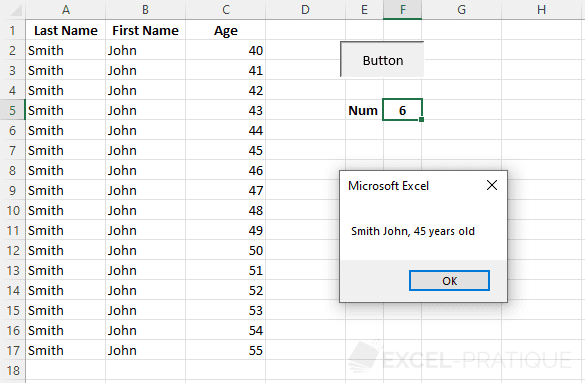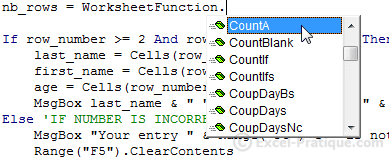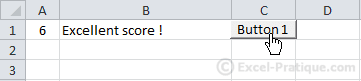VBA Course: Conditions

Conditions are very useful in programming because they allow us to execute actions based on specific criteria (it's the same principle as the IF function).

The most important conditional function is If, and now we'll take a look at how it works:

If [CONDITION HERE] Then ' => IF condition is validated, THEN
'Instructions if true
Else ' => OTHERWISE
'Instructions if false
End If

Let's move right on to practice and return to the example that we used in the lesson on variables. The purpose of this procedure was to display a dialog box containing the data from the row whose number is indicated in cell F5:

Source file: conditions.xlsIf you enter a letter in cell F5, it will cause a bug. We want to prevent that from happening.

Sub variables()
'Declaring variables
Dim last_name As String, first_name As String, age As Integer, row_number As Integer

'Assigning values to variables
row_number = Range("F5") + 1
last_name = Cells(row_number, 1)
first_name = Cells(row_number, 2)
age = Cells(row_number, 3)

'Dialog box
MsgBox last_name & " " & first_name & ", " & age & " years old"
End Sub

Let's begin by adding a condition that will verify that the value of cell F5 is numerical before the code is executed.

We'll use the function IsNumeric to test this condition:

Sub variables()

'If the value in parentheses (cell F5) is numerical (AND THEREFORE IF THE CONDITION IS TRUE) then
'execute the instructions that follow THEN
If IsNumeric(Range("F5")) Then

'Declaring variables
Dim last_name As String, first_name As String, age As Integer, row_number As Integer
'Values of variables
row_number = Range("F5") + 1
last_name = Cells(row_number, 1)
first_name = Cells(row_number, 2)
age = Cells(row_number, 3)
'Dialog Box
MsgBox last_name & " " & first_name & ", " & age & " years old"

End If

End Sub

We also need to add instructions to execute in case the conditions are not met:

Sub variables()

If IsNumeric(Range("F5")) Then 'IF CONDITION TRUE

'Declaring variables
Dim last_name As String, first_name As String, age As Integer, row_number As Integer
'Values of variables
row_number = Range("F5") + 1
last_name = Cells(row_number, 1)
first_name = Cells(row_number, 2)
age = Cells(row_number, 3)
'Dialog box
MsgBox last_name & " " & first_name & ", " & age & " years old"

Else 'IF CONDITION FALSE

'Dialog box: warning
MsgBox "Your entry" & Range("F5") & " is not valid !"
'Deleting the contents of cell F5
Range("F5").ClearContents

End If

End Sub

Now non-numerical values won't cause any problems.

Working with our array, which contains 16 rows of data, our next step will be to test whether the variable row_number: "greater than or equal to 2" and "less than or equal to 17".

But first, have a look at this list of comparison operators:

 = is equal to <> is different than < is less than <= is less than or eual to > is greater than >= is greater than or equal to

And these other useful operators:

 AND [condition1] AND [condition2]The two conditions must be true OR [condition1] OR [condition2]At least 1 of the 2 conditions must be true NOT NOT [condition1]The condition should be false

Now let's add the conditions that we mentioned above, using AND along with the comparison operators listed above:

Sub variables()
If IsNumeric(Range("F5")) Then 'IF NUMERICAL
Dim last_name As String, first_name As String, age As Integer, row_number As Integer
row_number = Range("F5") + 1

If row_number >= 2 And row_number <= 17 Then 'IF CORRECT NUMBER
last_name = Cells(row_number, 1)
first_name = Cells(row_number, 2)
age = Cells(row_number, 3)
MsgBox last_name & " " & first_name & ", " & age & " years old"
Else 'IF NUMBER IS INCORRECT
MsgBox "Your entry " & Range("F5") & " is not a valid number !"
Range("F5").ClearContents
End If

Else 'IF NOT NUMERICAL
MsgBox "Your entry " & Range("F5") & " is not valid !"
Range("F5").ClearContents
End If
End Sub

If we wanted to make our macro a bit more practical, we could replace 17 with a variable that contained the number of rows. This would let us add or remove lines from our array without having to change this limit each time.

In order to do this, we have to create a variable nb_rows and add this function:In this case, we'll use WorksheetFunction.CountA which is the function COUNTA which you may already be familiar with ...

We want this function to count the number of non-empty cells in the first column and then replace 17 with nb_rows:

Sub variables()
If IsNumeric(Range("F5")) Then 'IF NUMERICAL
Dim last_name As String, first_name As String, age As Integer, row_number As Integer
Dim nb_rows As Integer

row_number = Range("F5") + 1
nb_rows = WorksheetFunction.CountA(Range("A:A")) 'NBVAL Function

If row_number >= 2 And row_number <= nb_rows Then 'IF CORRECT NUMBER
last_name = Cells(row_number, 1)
first_name = Cells(row_number, 2)
age = Cells(row_number, 3)
MsgBox last_name & " " & first_name & ", " & age & " years old"
Else 'IF NUMBER IS INCORRECT
MsgBox "Your entry " & Range("F5") & " is not a valid number !"
Range("F5").ClearContents
End If

Else 'IF NOT NUMERICAL
MsgBox "Your entry " & Range("F5") & " is not valid !"
Range("F5").ClearContents
End If
End Sub

ElseIf

ElseIf makes it possible to add more conditions after the IF command:

If [CONDITION 1] Then ' => IF condition 1 is true, THEN
'Instructions 1
ElseIf [CONDITION 2] Then ' => IF condition 1 is false, but condition 2 is true, THEN
'Instructions 2
Else ' => OTHERWISE
'Instructions 3
End If

If condition 1 is true, Instructions 1 will be executed, and we will leave the If command (which begins with If and ends with End If). If condition 1 is false, we continue to condition 2. If this condition is true, Instructions 2 will be executed, and if it is false, then Instructions 3 (under Else) will be executed.

Here is an example, with a score between 1 and 6 in cell A1 (without decimals in this case) and a score_comment in cell B1 based on the score:

Sub scores_comment()
'Variables
Dim note As Integer, score_comment As String
note = Range("A1")

'Comments based on the score
If note = 6 Then
score_comment = "Excellent score !"
ElseIf note = 5 Then
score_comment = "Good score"
ElseIf note = 4 Then
score_comment = "Satisfactory score"
ElseIf note = 3 Then
score_comment = "Unsatisfactory score"
ElseIf note = 2 Then
score_comment = "Bad score"
ElseIf note = 1 Then
score_comment = "Terrible score"
Else
score_comment = "Zero score"
End If

Range("B1") = score_comment
End SubSelect

There is an alternative to using If with lots of ElseIf instructions: the Select command, which is better suited to these sorts of situations.

Here is an example of the same macro written with Select:

Sub scores_comment()
'Variables
Dim note As Integer, score_comment As String
note = Range("A1")

'Comments based on the score
Select Case note    ' <= the value to test (the score, in this case)
Case Is = 6         ' <= if the value = 6
score_comment = "Excellent score !"
Case Is = 5         ' <= if the value = 5
score_comment = "Good score"
Case Is = 4         ' <= if the value = 4
score_comment = "Satisfactory score"
Case Is = 3         ' <= if the value = 3
score_comment = "Unsatisfactory score"
Case Is = 2         ' <= if the value = 2
score_comment = "Bad score"
Case Is = 1         ' <= if the value = 1
score_comment = "Terrible score"
Case Else           ' <= if the value isn't equal to any of the above values
score_comment = "Zero score"
End Select

'Comment in B1
Range("B1") = score_comment
End Sub

Please note that we could also have used other comparison operators, for example:

Case Is >= 6         'if the value is >= 6

Examples with different values:

Case Is = 6, 7       'if the values is = 6 or 7
Case Is <> 6, 7      'if the value is different than 6 or 7
Case 6 To 10         'if the value is = any number between 6 and 10